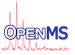OpenMS
BasicStatistics< RealT > Class Template Reference

Calculates some basic statistical parameters of a distribution: sum, mean, variance, and provides the normal approximation. More...

#include <OpenMS/MATH/STATISTICS/BasicStatistics.h>

Inheritance diagram for BasicStatistics< RealT >:
[legend]
Collaboration diagram for BasicStatistics< RealT >:
[legend]

## Public Types

typedef RealT RealType
The real type specified as template argument. More...

typedef std::vector< RealTypeprobability_container

typedef std::vector< RealTypecoordinate_container

## Public Member Functions

BasicStatistics ()
Default constructor. More...

BasicStatistics (BasicStatistics const &arg)
Copy constructor. More...

BasicStatisticsoperator= (BasicStatistics const &arg)
Assignment. More...

void clear ()
Set sum, mean, and variance to zero. More...

template<typename ProbabilityIterator >
void update (ProbabilityIterator probability_begin, ProbabilityIterator const probability_end)
This does the actual calculation. More...

template<typename ProbabilityIterator , typename CoordinateIterator >
void update (ProbabilityIterator const probability_begin, ProbabilityIterator const probability_end, CoordinateIterator const coordinate_begin)
This does the actual calculation. More...

RealType mean () const
Returns the mean. More...

void setMean (RealType const &mean)

RealType variance () const
Returns the variance. More...

void setVariance (RealType const &variance)

RealType sum () const
Returns the sum. More...

void setSum (RealType const &sum)

RealType normalDensity_sqrt2pi (RealType coordinate) const
Returns the density of the normal approximation at point, multiplied by sqrt( 2 * pi ). This saves a division operation compared to normalDensity() More...

RealType normalDensity (RealType const coordinate) const
See normalDensity_sqrt2pi(). Returns the density of the normal distribution at point. More...

void normalApproximation (probability_container &probability)
The argument probability is filled with values according to the normal approximation. Its size() is not changed. The approximation takes place at coordinate positions 0, 1, ..., size()-1. More...

void normalApproximation (probability_container &probability, typename probability_container::size_type const size)

void normalApproximation (probability_container &probability, coordinate_container const &coordinate)
The argument probability is filled with values according to the normal approximation. The second argument coordinate contains the positions where the approximation takes place. probability.size() is set to coordinate.size(). More...

## Static Public Member Functions

static RealType sqrt2pi ()
Returns sqrt( 2 * pi ), which is useful to normalize the result of normalDensity_sqrt2pi(). More...

## Protected Attributes

Protected Members
RealType mean_

RealType variance_

RealType sum_

## Private Member Functions

Private Methods
void normalApproximationHelper_ (probability_container &probability, typename probability_container::size_type const size)

void normalApproximationHelper_ (probability_container &probability, coordinate_container const &coordinate)

## Related Functions

(Note that these are not member functions.)

std::ostream & operator<< (std::ostream &os, BasicStatistics &arg)
A convenient overload for debugging purposes. More...

## Detailed Description

### template<typename RealT = double> class OpenMS::Math::BasicStatistics< RealT >

Calculates some basic statistical parameters of a distribution: sum, mean, variance, and provides the normal approximation.

The intended usage is as follows:

• create an instance
• set the basic statistical parameters by either
• calling one of the update() member functions, or
• using the set... methods
• do something with the basic statistical parameters, e.g.
• using the get... methods, or
• obtain samples from a normal approximation with these parameters
• whatever member function you might want to add to this class ;-)

## ◆ coordinate_container

 typedef std::vector coordinate_container

## ◆ probability_container

 typedef std::vector probability_container

## ◆ RealType

 typedef RealT RealType

The real type specified as template argument.

## ◆ BasicStatistics() [1/2]

 BasicStatistics ( )
inline

Default constructor.

## ◆ BasicStatistics() [2/2]

 BasicStatistics ( BasicStatistics< RealT > const & arg )
inline

Copy constructor.

## ◆ clear()

 void clear ( )
inline

Set sum, mean, and variance to zero.

## ◆ mean()

 RealType mean ( ) const
inline

Returns the mean.

## ◆ normalApproximation() [1/3]

 void normalApproximation ( probability_container & probability )
inline

The argument probability is filled with values according to the normal approximation. Its size() is not changed. The approximation takes place at coordinate positions 0, 1, ..., size()-1.

## ◆ normalApproximation() [2/3]

 void normalApproximation ( probability_container & probability, coordinate_container const & coordinate )
inline

The argument probability is filled with values according to the normal approximation. The second argument coordinate contains the positions where the approximation takes place. probability.size() is set to coordinate.size().

## ◆ normalApproximation() [3/3]

 void normalApproximation ( probability_container & probability, typename probability_container::size_type const size )
inline

The argument probability is filled with values according to the normal approximation. Its size() is set to size. The approximation takes place at coordinate positions 0, 1, ..., size-1.

## ◆ normalApproximationHelper_() [1/2]

 void normalApproximationHelper_ ( probability_container & probability, coordinate_container const & coordinate )
inlineprivate

References OpenMS::Math::sum().

## ◆ normalApproximationHelper_() [2/2]

 void normalApproximationHelper_ ( probability_container & probability, typename probability_container::size_type const size )
inlineprivate

References OpenMS::Math::sum().

## ◆ normalDensity()

 RealType normalDensity ( RealType const coordinate ) const
inline

See normalDensity_sqrt2pi(). Returns the density of the normal distribution at point.

## ◆ normalDensity_sqrt2pi()

 RealType normalDensity_sqrt2pi ( RealType coordinate ) const
inline

Returns the density of the normal approximation at point, multiplied by sqrt( 2 * pi ). This saves a division operation compared to normalDensity()

References OpenMS::Math::mean(), and OpenMS::Math::variance().

## ◆ operator=()

 BasicStatistics& operator= ( BasicStatistics< RealT > const & arg )
inline

Assignment.

## ◆ setMean()

 void setMean ( RealType const & mean )
inline

References OpenMS::Math::mean().

## ◆ setSum()

 void setSum ( RealType const & sum )
inline

References OpenMS::Math::sum().

## ◆ setVariance()

 void setVariance ( RealType const & variance )
inline

## ◆ sqrt2pi()

 static RealType sqrt2pi ( )
inlinestatic

Returns sqrt( 2 * pi ), which is useful to normalize the result of normalDensity_sqrt2pi().

## ◆ sum()

 RealType sum ( ) const
inline

Returns the sum.

## ◆ update() [1/2]

 void update ( ProbabilityIterator const probability_begin, ProbabilityIterator const probability_end, CoordinateIterator const coordinate_begin )
inline

This does the actual calculation.

You can call this as often as you like, using different input vectors.

## ◆ update() [2/2]

 void update ( ProbabilityIterator probability_begin, ProbabilityIterator const probability_end )
inline

This does the actual calculation.

You can call this as often as you like, using different input vectors.

Referenced by AsymmetricStatistics< RealT >::update().

## ◆ variance()

 RealType variance ( ) const
inline

Returns the variance.

## ◆ operator<<

 std::ostream& operator<< ( std::ostream & os, BasicStatistics< RealT > & arg )
friend

A convenient overload for debugging purposes.

## ◆ mean_

 RealType mean_
protected

## ◆ sum_

 RealType sum_
protected

## ◆ variance_

 RealType variance_
protected